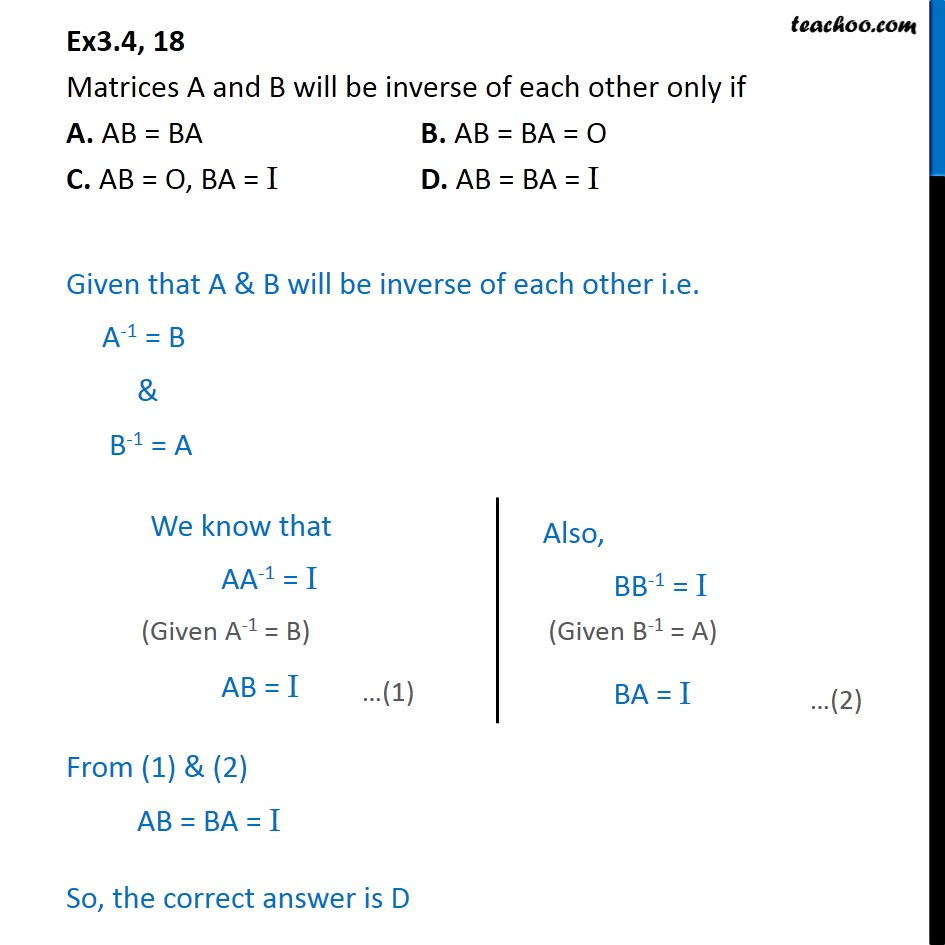Inverse of matrix using elementary transformation

Chapter 3 Class 12 Matrices
Concept wiseIntroducing your new favourite teacher - Teachoo Black, at only ₹83 per month

### Transcript

Ex3.4, 18 Matrices A and B will be inverse of each other only if A. AB = BA B. AB = BA = O C. AB = O, BA = I D. AB = BA = I Given that A & B will be inverse of each other i.e. A-1 = B & B-1 = A From (1) & (2) AB = BA = I So, the correct answer is D